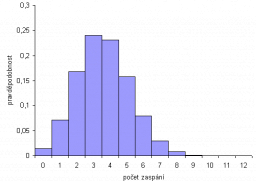# Bernoulli trial

A used car saleswoman estimates that each time she shows a customer a car, there is a probability of 0.1 that the customer will buy the car. The saleswoman would like to sell at least one car per week. If showing a car is a Bernoulli trial with a probability of 0.95 of at least one sale,
(i) how many cars would the saleswoman show per week?
(ii) calculate the probability of exactly three sales per week.

p1 =  0

### Step-by-step explanation:Did you find an error or inaccuracy? Feel free to write us. Thank you!

Tips for related online calculators
Looking for a statistical calculator?
Would you like to compute the count of combinations?